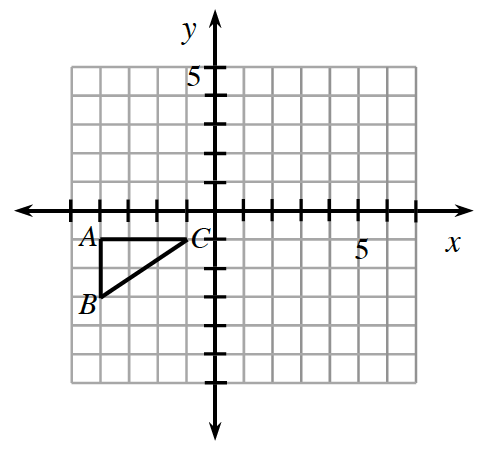### Home > CC3 > Chapter 8 > Lesson 8.3.1 > Problem8-135

8-135.This problem is a checkpoint for transformations. It will be referred to as Checkpoint 8.

$ΔABC$ at right is transformed into $ΔXYZ$. Tell the coordinates of the vertices of the new triangle after each of the following transformations.

1. Translate $ΔABC$  $2$ units right and $3$ units up.

2. Reflect $ΔABC$ across the $y$-axis.

3. Rotate $ΔABC$  $90°$clockwise about the origin.

4. Dilate $ΔABC$ with a scale factor of $2$ from the origin.Check your answers by referring to the Checkpoint 8 materials located at the back of your book.

Ideally, at this point you are comfortable working with these types of problems and can solve them correctly. If you feel that you need more confidence when solving these types of problems, then review the Checkpoint 8 materials and try the practice problems provided. From this point on, you will be expected to do problems like these correctly and with confidence.

Answers and extra practice for the Checkpoint problems are located in the back of your printed textbook or in the Reference Tab of your eBook. If you have an eBook for Core Connections, Course 3, login and then click the following link: Checkpoint 8: Transformations